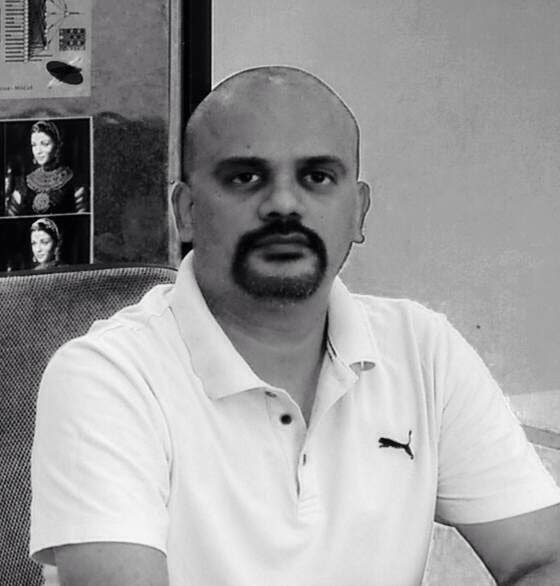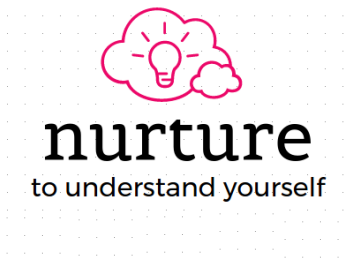Asst. Professor of Mathematics
Department of Mathematics

Main Office: CLC 1b, Central University of Tamil Nadu, Thiruvarur, Neelakudy, Tamil Nadu 610005
Phone: +91-8300251729 / +91-9962551729
Email: vpramesh@gmail.com, rameshmat@cutn.ac.in

Our Pedagogical InitiativeWe are sharing a few e-learning materials developed by us. Please feel free to let us know your feedback/suggestions.

Algebra / Number Theory

• The Symmetric group S_n and Sudoku Problem (Complexity of Sudoku solving algorithms - a new theory) [pdf]
• Algorithms for generating all possible combinations in lexicographic order [pdf]

Computability and Complexity

• Artificial Neural Network - Design and Analysis of back propagation Algorithm [pdf]
• Computability of Decision Problems [pdf]
• Time Complexity Analysis of Turing Machines [pdf]

Scientific Computing using Open Source software

• Introduction to Big Data Analytics and a few Open Source Software [pdf]
• Regression- its application and computation using R and Python [pdf]

Open Source Software - R:

• Introduction to Computing using R [pdf]
• Statistical Computing using R [pdf]
• Computational Number theory using R [pdf]
• Data Visualization (Graphics) using R [pdf]

Open Source Software - Python:

• Introduction to Computing using Python [pdf]
• Statistical Computing using Python [pdf]
• Computational Number theory using Python [pdf]
• Data Visualization (Graphics) using Python [pdf]

Case Studies:

• Case Study 1: Healthcare Big Data Analytics [pdf]
• Case Study 2: Financial Big Data Analytics
• Case Study 3: Consumer Big Data Analytics [pdf]